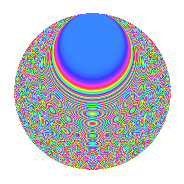Properties

 Label 8026.2.aLevel 8026 Weight 2 Character orbit a Rep. character $$\chi_{8026}(1,\cdot)$$ Character field $$\Q$$ Dimension 334 Newforms 4 Sturm bound 2007 Trace bound 1

Related objects

Defining parameters

 Level: $$N$$ = $$8026 = 2 \cdot 4013$$ Weight: $$k$$ = $$2$$ Character orbit: $$[\chi]$$ = 8026.a (trivial) Character field: $$\Q$$ Newforms: $$4$$ Sturm bound: $$2007$$ Trace bound: $$1$$

Dimensions

The following table gives the dimensions of various subspaces of $$M_{2}(\Gamma_0(8026))$$.

Total New Old
Modular forms 1005 334 671
Cusp forms 1002 334 668
Eisenstein series 3 0 3

The following table gives the dimensions of the cuspidal new subspaces with specified eigenvalues for the Atkin-Lehner operators and the Fricke involution.

$$2$$$$4013$$FrickeDim.
$$+$$$$+$$$$+$$$$81$$
$$+$$$$-$$$$-$$$$86$$
$$-$$$$+$$$$-$$$$96$$
$$-$$$$-$$$$+$$$$71$$
Plus space$$+$$$$152$$
Minus space$$-$$$$182$$

Trace form

 $$334q$$ $$\mathstrut +\mathstrut 334q^{4}$$ $$\mathstrut +\mathstrut 4q^{5}$$ $$\mathstrut -\mathstrut 2q^{6}$$ $$\mathstrut +\mathstrut 328q^{9}$$ $$\mathstrut +\mathstrut O(q^{10})$$ $$334q$$ $$\mathstrut +\mathstrut 334q^{4}$$ $$\mathstrut +\mathstrut 4q^{5}$$ $$\mathstrut -\mathstrut 2q^{6}$$ $$\mathstrut +\mathstrut 328q^{9}$$ $$\mathstrut +\mathstrut 6q^{10}$$ $$\mathstrut +\mathstrut 2q^{11}$$ $$\mathstrut -\mathstrut 2q^{13}$$ $$\mathstrut +\mathstrut 334q^{16}$$ $$\mathstrut +\mathstrut 16q^{17}$$ $$\mathstrut -\mathstrut 10q^{19}$$ $$\mathstrut +\mathstrut 4q^{20}$$ $$\mathstrut -\mathstrut 4q^{22}$$ $$\mathstrut +\mathstrut 12q^{23}$$ $$\mathstrut -\mathstrut 2q^{24}$$ $$\mathstrut +\mathstrut 340q^{25}$$ $$\mathstrut +\mathstrut 4q^{26}$$ $$\mathstrut -\mathstrut 24q^{30}$$ $$\mathstrut -\mathstrut 4q^{31}$$ $$\mathstrut -\mathstrut 20q^{33}$$ $$\mathstrut -\mathstrut 8q^{34}$$ $$\mathstrut +\mathstrut 4q^{35}$$ $$\mathstrut +\mathstrut 328q^{36}$$ $$\mathstrut -\mathstrut 4q^{37}$$ $$\mathstrut -\mathstrut 16q^{38}$$ $$\mathstrut -\mathstrut 56q^{39}$$ $$\mathstrut +\mathstrut 6q^{40}$$ $$\mathstrut +\mathstrut 12q^{41}$$ $$\mathstrut -\mathstrut 6q^{43}$$ $$\mathstrut +\mathstrut 2q^{44}$$ $$\mathstrut +\mathstrut 20q^{45}$$ $$\mathstrut -\mathstrut 8q^{46}$$ $$\mathstrut +\mathstrut 16q^{47}$$ $$\mathstrut +\mathstrut 346q^{49}$$ $$\mathstrut +\mathstrut 8q^{50}$$ $$\mathstrut -\mathstrut 16q^{51}$$ $$\mathstrut -\mathstrut 2q^{52}$$ $$\mathstrut +\mathstrut 10q^{53}$$ $$\mathstrut -\mathstrut 20q^{54}$$ $$\mathstrut +\mathstrut 32q^{55}$$ $$\mathstrut -\mathstrut 20q^{57}$$ $$\mathstrut +\mathstrut 10q^{58}$$ $$\mathstrut -\mathstrut 6q^{59}$$ $$\mathstrut -\mathstrut 22q^{61}$$ $$\mathstrut +\mathstrut 8q^{62}$$ $$\mathstrut -\mathstrut 44q^{63}$$ $$\mathstrut +\mathstrut 334q^{64}$$ $$\mathstrut -\mathstrut 4q^{65}$$ $$\mathstrut -\mathstrut 16q^{66}$$ $$\mathstrut -\mathstrut 14q^{67}$$ $$\mathstrut +\mathstrut 16q^{68}$$ $$\mathstrut -\mathstrut 28q^{69}$$ $$\mathstrut +\mathstrut 20q^{70}$$ $$\mathstrut +\mathstrut 4q^{73}$$ $$\mathstrut +\mathstrut 10q^{74}$$ $$\mathstrut -\mathstrut 28q^{75}$$ $$\mathstrut -\mathstrut 10q^{76}$$ $$\mathstrut +\mathstrut 16q^{77}$$ $$\mathstrut +\mathstrut 20q^{78}$$ $$\mathstrut -\mathstrut 20q^{79}$$ $$\mathstrut +\mathstrut 4q^{80}$$ $$\mathstrut +\mathstrut 294q^{81}$$ $$\mathstrut +\mathstrut 16q^{82}$$ $$\mathstrut -\mathstrut 2q^{83}$$ $$\mathstrut -\mathstrut 4q^{85}$$ $$\mathstrut -\mathstrut 8q^{86}$$ $$\mathstrut -\mathstrut 4q^{87}$$ $$\mathstrut -\mathstrut 4q^{88}$$ $$\mathstrut +\mathstrut 4q^{89}$$ $$\mathstrut +\mathstrut 30q^{90}$$ $$\mathstrut +\mathstrut 4q^{91}$$ $$\mathstrut +\mathstrut 12q^{92}$$ $$\mathstrut +\mathstrut 8q^{93}$$ $$\mathstrut +\mathstrut 4q^{94}$$ $$\mathstrut -\mathstrut 28q^{95}$$ $$\mathstrut -\mathstrut 2q^{96}$$ $$\mathstrut -\mathstrut 4q^{97}$$ $$\mathstrut +\mathstrut 46q^{99}$$ $$\mathstrut +\mathstrut O(q^{100})$$

Decomposition of $$S_{2}^{\mathrm{new}}(\Gamma_0(8026))$$ into irreducible Hecke orbits

Label Dim. $$A$$ Field CM Traces A-L signs $q$-expansion
$$a_2$$ $$a_3$$ $$a_5$$ $$a_7$$ 2 4013
8026.2.a.a $$71$$ $$64.088$$ None $$71$$ $$-9$$ $$-34$$ $$-19$$ $$-$$ $$-$$
8026.2.a.b $$81$$ $$64.088$$ None $$-81$$ $$-10$$ $$-26$$ $$3$$ $$+$$ $$+$$
8026.2.a.c $$86$$ $$64.088$$ None $$-86$$ $$11$$ $$25$$ $$-3$$ $$+$$ $$-$$
8026.2.a.d $$96$$ $$64.088$$ None $$96$$ $$8$$ $$39$$ $$19$$ $$-$$ $$+$$

Decomposition of $$S_{2}^{\mathrm{old}}(\Gamma_0(8026))$$ into lower level spaces

$$S_{2}^{\mathrm{old}}(\Gamma_0(8026)) \cong$$ $$S_{2}^{\mathrm{new}}(\Gamma_0(4013))$$$$^{\oplus 2}$$